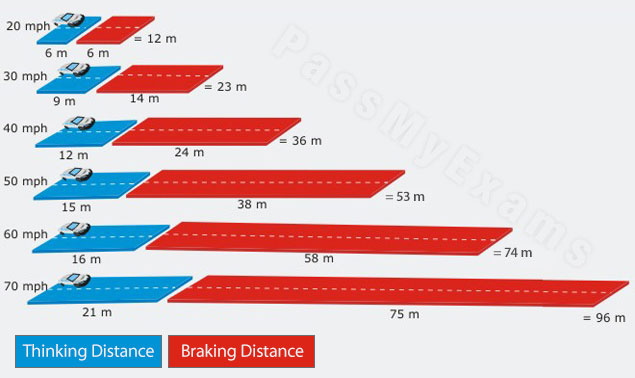## Stopping Distance

Cars rely on friction at the brakes and tyres in order to stop.

The total distance a car requires to stop is called the total stopping distance. This is the sum of the distance covered in the time it takes for the driver to react known as the thinking distance and the distance the car travels before coming to rest after the brakes are pressed called the braking distance.

Total stopping distance = thinking distance + braking distance

The diagram below gives the shortest stopping distances for a car on a dry road with good brakes and tyres:From the diagram a clear pattern can be seen between the velocity of the car and the total stopping distance. The average reaction time of a driver is about 0.7s as the velocity of the car increases so will the thinking distance from the relationship:

Distance travelled = velocity x reaction time

The braking distances increases because at a faster velocity the car possesses more kinetic energy. This energy has to be transferred to the brakes; this is transferred as heat and is the reason why brakes become hot. The relationship between velocity and kinetic energy is:

Kinetic Energy (Joules) = ½ x mass x velocity2

Therefore if the car travels three times the velocity it has 9 times the kinetic energy which means the braking distance will be nine times longer.

## Factors affecting the total stopping distance

### The driver’s reaction time

The thinking distance depends on the driver’s reaction time. Reactions are strongly influenced by the state of the mind. Thus, a driver under the influence of a drug such as alcohol will have a much reduced reaction time which will increase the thinking distance. Tiredness and fatigue also influence reaction time. A tired driver will react a lot slower than an alert driver.

### Velocity

As illustrated in the chart above the braking distance increases with velocity. This is explained by the relationship between kinetic energy and velocity. The greater the velocity the greater the kinetic energy the brakes have to transfer.

### Mass

The mass of the vehicle is also related to kinetic energy in the relationship;

Kinetic Energy (Joules) = ½ x mass x velocity2

The greater the mass the greater the kinetic energy, thus a heavier car will require a longer braking distance.